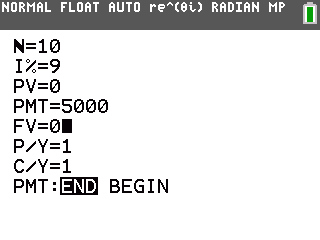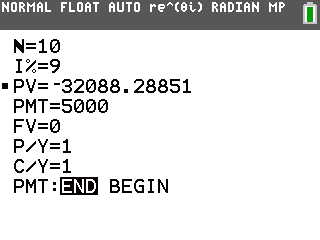# Knowledge Base

## Solution 34832: Calculating the Present Value of an Annuity on a TI-84 Plus C Silver Edition.

### How do I calculate the present value of an annuity on a TI-84 Plus C Silver Edition?

A regular annuity is a series of equal cash flows occurring at equally spaced time periods. In a regular annuity, the first cash flow occurs at the end of the first period.

Suppose that a client is offered an investment that will pay $5,000 per year for 10 years. If the client can earn a rate of 9% per year on similar investments, how much should the client be willing to pay for this annuity? To calculate above example please follow steps listed below: • First, access the TVM solver by pressing [APPS]. • Press 1:Finance. • Press 1:TVM Solver. • Press   [↓]  [↓]  [↓]     [↓]  [↓]  [↑].•Next press [↑] [↑] [ALPHA] [SOLVE] to calculate the present value:The present value is$-32,088.29.